Follow

# Displaying Logarithmically Distributed Data in Surfer

Surfer now supports gridding data using a logarithmic scale, and displaying logarithmically distributed contour levels and color scales! This is extremely useful when your Z data spans several orders of magnitude, such as concentration data where the Z values can range from very small values (i.e. 0.001) to very large values (i.e. >10000). In such cases, gridding with a linear scale biases the grid to the high Z values and thus looses detail in areas with the lower Z values.

Gridding and displaying the data on a logarithmic scale allows you to better discern the variability in the lower and higher data ranges. The extreme high and low values are not smoothed over, as they are when gridded and displayed using a linear scale. There are a number of features to display logarithmically distributed data (starting in Surfer 12). This article will go through these options.

Gridding Data

If you have logarithmically distributed data, the first step is to grid the data with a logarithmic transform.

1. Click Home | Grid Data | Grid Data in Surfer.
2. Select your data file and click Open.
3. Choose the appropriate Z Transform option in the lower right corner of the Grid Data dialog. You can choose:
1. Linear. This is the default, and how all previous versions of Surfer gridded data. It will treat the Z value in the data as linear, grid the linear values, and save the linear Z value back to the grid file. If your data is not logarithmically distributed (i.e. the Z value in the data does not span multiple orders of magnitude), choose this option.
2. Log, save as log. This will automatically take the log of the Z values in the data file, grid the log values, and save the grid. The Z of the grid is the log of the original data in the data file. Choose this option if your data spans several orders of magnitude and you want the resulting Z value in the grid file to be in logarithmic units.
3. Log, save as linear. This will automatically take the log of the Z values in the data file, grid the log values, convert the log values in the grid back to linear values, and save the grid with the Z being in the original linear data units. Choose this option if your data spans several orders of magnitude and you want the resulting Z value in the grid file to be in the same units as your original data.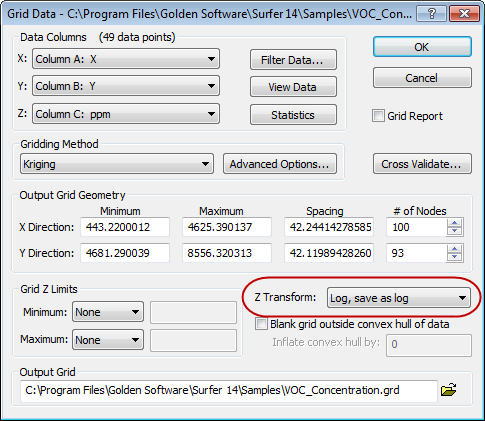Choose to transform the Z to take the log of the data automatically when you grid the data.
4. Set any other gridding parameters you wish.
5. Click OK to create the grid file.

Displaying Data

Once the grid is created, you can create maps from it, such as contour, color relief or 3D surface maps. How you set the contour level method or the colormap properties will depend on the Z transform chosen when gridding the data.

Linear and Log, save as log

If you grid the data with a Z Transform of Linear or Log, save as log, display contours with the Simple or Advanced level methods. Display other map types (i.e. color relief, shaded relief, or 3D surfaces) with the regular linearly-scaled colormap. You do not need to do anything specific when producing a map from a grid file created using one of these Z Transform methods.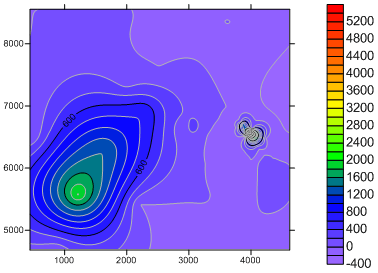The above map shows a contour map created from a grid with Z Transform set to Linear. It shows the linear distribution of the data. This map is shown using the default Simple contour level method. However, the areas with the very low data values and the areas with the very high data values are smoothed over.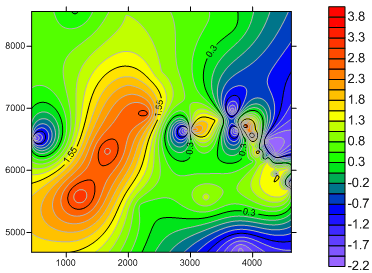The above map shows a contour map created from a grid with Z Transform set to Log, save as log. This map is shown using the default Simple contour level method and the Z values (as seen on the color scale) are in log units. This map shows the log-distributed data much better than the linear contour map above, as it more closely honors the very low and very high data points.

Log, save as linear

If you grid the data with a Z Transform of Log, save as linear, display contours with the Logarithmic level method. Display other map types (i.e. color relief or 3D surface maps) with the logarithmically-scaled colormap. This will give you a map showing the logarithmic distribution of the data, but with the Z values of your original data. The options to choose for map display depend on the type of map created.

Contour Map

Create a contour map with logarithmic contours by following these steps:

1. Click Home | New Map | Contour, select the grid file created with the Z Transform set to Log, save as linear and click Open.
2. Select the Contours layer in the Contents window.
3. In the Properties window, on the Levels page, change the Level Method from Simple to Logarithmic. The contour levels and the colormap are both automatically scaled logarithmically.
4. You can customize the map by changing other options, such as the Minor levels per decade option to control the number of minor contour lines between decades.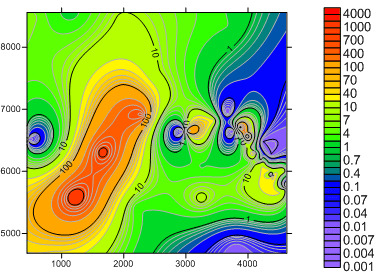The above shows a contour map created from a grid with Z Transform set to Log, save as linear. This map is shown using the Logarithmic contour level method and the Z values (as seen on the color scale) are in the original data units. It shows the log-distributed data much better than the linear contour map, as it more closely honors the very low and very high data areas.

Other Map Types (i.e. Color Relief or 3D Surface Maps)

If you do not want to create a contour map, you can still display logarithmically distributed colors for the other map types. If you do not scale the colors logarithmically, they will be assigned to the Z values based on the linear interpolation of the data values, thus skewing the colors to the higher Z values.

To assign a logarithmically scaled colormap:

1. Click Home | New Map | Color Relief, select the grid file created using the Log, save as linear Z transform and click Open.
2. Select the Color Relief layer in the Contents window.
3. In the Properties window, on the General page, click the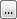button to the right of Colors.
4. In the Colormap dialog check the Logarithmic scaling check box. You will not see the nodes on the colormap change position, but if you select a node you will see that the Value for each node is now calculated on a log scale.
5. You might also want to check Use data limits.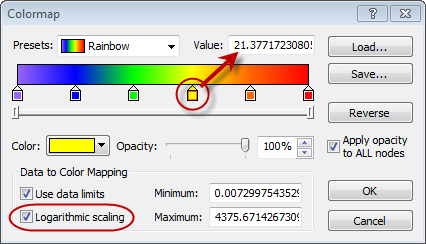Check the Logarithmic scaling check box to create a logarithmic distribution for the colormap. The Value for each of the color nodes updates according to the new scale. For example, the yellow node initially had a value of 2625.406 on a linear scale and it was converted to 21.377 on a logarithmic scale.
6. Click OK. The map is updated with the new color scale.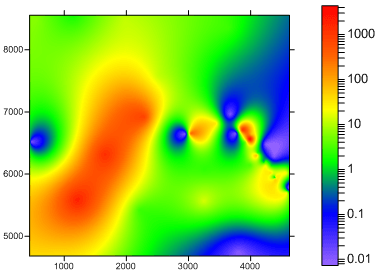The above map shows a color relief map created from a grid with Z Transform set to Log, save as linear, and is displayed with Logarithmic scaling enabled for the colormap.

An example data file you can start to experiment with is VOC_Concentration.xlsx in the Surfer Samples folder (by default C:\Program Files\Golden Software\Surfer 14). The above three contour map examples can be found in the sample file Logarithmic.srf. If there are any questions about gridding or displaying logarithmic data in Surfer 14, please email surfersupport@goldensoftware.com.

FAQs:

1. Using the Logarithmic contour level method, how are the minor contours created? They appear to be linear.

When the Level method is set to Logarithmic, the major contour lines occur at the decades (0.1, 1, 10, 100, 1000, etc.). The distance from 1 to 10 is the same as the distance from 10 to 100. This is a proper logarithmic scaling with log values of equal spacing.

You then have the option to specify the number of minor contour levels per decade (the default is 8). So between the major contour lines at 1 and 10, consider the 8 minor levels at 2, 3, 4 , 5, 6, 7, 8, and 9. These are not the same distance apart. The distance from 9 to 10 is much smaller than the distance from 1 to 2. The actual numbers (2, 3, 4, 5, 6, 7, 8, 9) are linear, but what makes them logarithmic is the unequal spacing between them.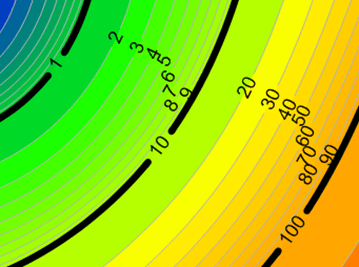The major contour lines at the decades are equally spaced, and the minor contour lines are linear values that are logarithmically spaced.
2. What If my grid file is already created? Can I still display it with logarithmic contour levels?

Yes, you can display any grid created linearly (i.e. a grid file created in a previous version of Surfer) with the contour level method set to Logarithmic. Depending on the Z range of the grid, that could give you a similar result to the Log, save as linear option with logarithmic contour levels, but not quite the same.

By gridding the data linearly, some of the extreme low and high values may have already been smoothed over. In addition, any negative or 0 values in the linear grid cannot be displayed with logarithmic contour levels and so extra steps should be taken to convert the negative or 0 data to a number >0.0.

Displaying a grid created with a linear scale as a contour map with logarithmic contours may be sufficient if you do not want to regrid the data or do not have the original data to regrid; but if you have logarithmically distributed data, it is most accurate to grid the log of the data.

3. How are the logarithmically scaled node values in the Colormap dialog calculated? In the example above, the log of 2625.406 is not 21.377.

The new nodal values in a logarithmically scaled colormap are calculated by taking the log of the current extents (the minimum and maximum of the colormap), calculating the log value of the node in the same position along the colormap as it was originally, and then taking the antilog of that value.

For example:
1. Take the log of the current extents. The data extents of the colormap are 0.007299754353 to 4375.671427. Take the log of both these values to calculate the log extents of -2.136691754 to 3.641044703.
2. Find the position of the node along the colormap. The yellow node is at the 60% position. You can find this out by looking at the CLR file (if you saved the colormap to a file) or by simply calculating it. To calculate it, take the existing node value, 2625.406, and divide it by the range of data (4375.671427 - 0.007299754353), and multiply by 100.
3. The 60% position between the calculated log extents (-2.136691754 to 3.641044703) is 1.3299485.
4. The antilog of 1.3299485 is 21.377. This is the new node value on the log scaled colormap.

4. I create a contour map and set the colormap to use logarithmic scaling, but almost the entire color scale is one color. What am I doing wrong?

For a contour map, the only time you should set the colormap to use logarithmic scaling is when the Level method for the contours layer is set to Logarithmic. If you use the Simple contour level method, and a logarithmically scaled colormap, then most of the colors will be squished at one end of the color scale. Select the contour map, click the Levels tab in the Properties window, and change the Level method to Logarithmic.

5. A lot of my data have negative numbers, but I keep getting an error that I need positive values. Why?

You cannot take the log of a negative number or 0. The number must be positive and non-zero. This is not Surfer's rule, but a mathematical one. The log of (X) becomes asymptotic as X approaches 0.

If you already have a grid file with negative or 0 values for some grid nodes, create a contour map, and try to set the Level method to Logarithmic, then you will get an error that the minimum contour level must be greater than 0.0. Click OK in the error. You will need to change the Minimum contour value to be >0.0, and then you can change the Level method to Logarithmic.

If you already have a grid file with negative or 0 values for some grid nodes, create a color relief map (or other map type) and try to change the colormap to use logarithmic scaling, then you will get an error that logarithmic scaling requires positive data. Click OK in the error. You will need to change the Minimum and/or Maximum data value for the colormap so that they are both >0.0.

6. What happens to the negative or 0 data when I grid the data with a log transform?

You cannot take the log of a negative number or 0. The number must be positive and non-zero. This is not Surfer's rule, but a mathematical one. The log of (X) becomes asymptotic as X approaches 0.

If you are gridding data that contains negative or 0 values, then these data points will be ignored if you are gridding with the Z Transform set to either Log, save as log or Log, save as linear.

Updated November 12, 2018